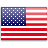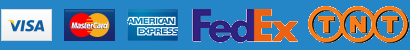Free shipping on all orders over \$ 500

# AbMole Molarity Calculator Calculate the mass, volume or concentration required for a solution

The AbMole molarity calculator is based on the following equation:
Mass (g) = Concentration (mol/L) x Volume (L) x Molecular Weight (g/mol)

Mass   Concentration   Volume   MW*
=   x   x

*When preparing stock solutions, please always use the batch-specific molecular weight of the product found on the vial label and MSDS / COA (available on product pages).

The AbMole molarity calculator is a useful tool which allows you to calculate the:

• mass of a compound required to prepare a solution of known volume and concentration
• volume of solution required to dissolve a compound of known mass to a desired concentration
• concentration of a solution resulting from a known mass of compound in a specific volume

##### An example of a molarity calculation using the AbMole molarity calculator

What is the mass of compound required to make a 10 mM stock solution in 10 ml of water given
that the molecular weight of the compound is 197.13 g/mol?

1. Enter 197.13 into the Molecular Weight (MW) box
2. Enter 10 into the Concentration box and select the correct unit (millimolar)
3. Enter 10 into the Volume box and select the correct unit (milliliter)
4. Press Calculate button
5. The answer of 19.713 mg appears in the Mass box

##### Resources